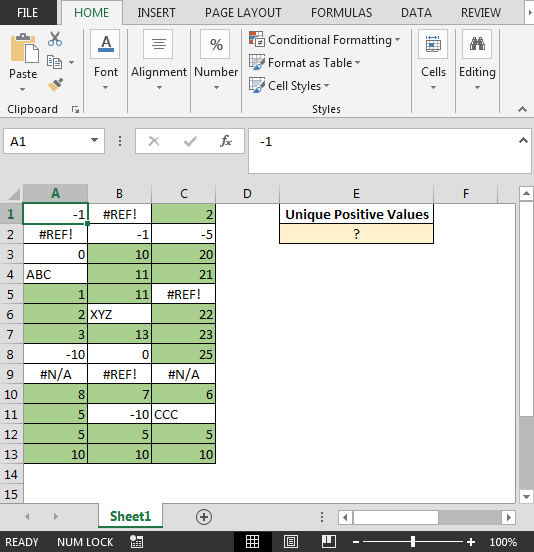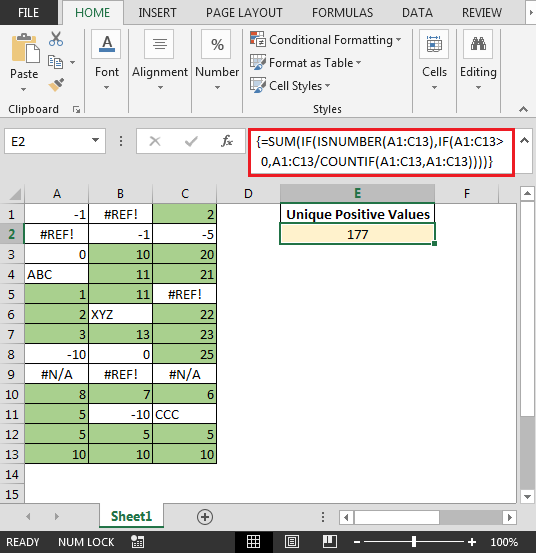# Excel Sum Only Positive Numbers

In this article, you will learn how to sum only unique positive values in Excel.

Question: I am working on importing Excel file which contains text, negative values, positive values or any errors.

• I want to sum unique positive values from data
• The formula should ignore any text, negative values or any error & return the sum of positive values.
• Example: If positive values are 1,1,5,5 & 10 then the result would be 16 i.e. (1+5+10)

The positive values have been highlighted in green color. Following is the snapshot of data:• In cell E2, the formula would be {=SUM(IF(ISNUMBER(A1:C13),IF(A1:C13>0,A1:C13/COUNTIF(A1:C13,A1:C13))))}

Note: This is an array formula. Hence, use CTRL + SHIFT + ENTER shortcut keys together.The above formula will add only the unique positive numbers.

In this way we can add unique positive values with combination of SUM, IF, ISNUMBER & COUNTIF functions.

Terms and Conditions of use

The applications/code on this site are distributed as is and without warranties or liability. In no event shall the owner of the copyrights, or the authors of the applications/code be liable for any loss of profit, any problems or any damage resulting from the use or evaluation of the applications/code.